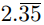Friday, October 22, 2021
Home > CBSE Class 10 > Extra MCQ Questions for Class 10 Maths Chapter 1 Real Numbers

# Extra MCQ Questions for Class 10 Maths Chapter 1 Real NumbersHi students, Welcome to AMBiPi (Amans Maths Blogs). In this article, you will get Extra MCQ Questions for Class 10 Maths Chapter 1 Real Numbers. You can download this PDF and save it in your mobile device or laptop etc.

Extra MCQ Questions for Class 10 Maths Real Numbers Question No 31:

225 can be expressed as

Option A : 5 × 32

Option B : 52 × 3

Option C : 52 × 32

Option D : 53 × 3

Option C : 52 × 32

Extra MCQ Questions for Class 10 Maths Real Numbers Question No 32:

If two positive integers p and q can be expressed as p = ab2 and q = a3 b; a, b being prime numbers, then LCM (p, q) is

Option A : ab

Option B : a2b2

Option C : a3b2

Option D : a3b3

Option C : a3b2

Extra MCQ Questions for Class 10 Maths Real Numbers Question No 33:

There are 312, 260 and 156 students in class X, XI and XII respectively. Buses are to be hired to take these students to a picnic. Find the maximum number of students who can sit in a bus if each bus takes equal number of students

Option A : 52

Option B : 56

Option C : 48

Option D : 63

Option A : 52

Extra MCQ Questions for Class 10 Maths Real Numbers Question No 34:is

Option A : an integer

Option B : a rational number

Option C : an irrational number

Option D : a natural number

Option B : a rational number

Extra MCQ Questions for Class 10 Maths Real Numbers Question No 35:

There is a circular path around a sports field. Priya takes 18 minutes to drive one round of the field. Harish takes 12 minutes. Suppose they both start at the same point and at the same time and go in the same direction. After how many minutes will they meet ?

Option A : 36 minutes

Option B : 18 minutes

Option C : 6 minutes

Option D : They will not meet each other.

Option A : 36 minutes

Extra MCQ Questions for Class 10 Maths Real Numbers Question No 36:

The least number that is divisible by all the numbers from 1 to 10 (both inclusive) is

Option A : 10

Option B : 100

Option C : 504

Option D : 2520

Option D : 2520

Extra MCQ Questions for Class 10 Maths Real Numbers Question No 37:

The values of x and y in the given figure areOption A : 7, 13

Option B : 13, 7

Option C : 9, 12

Option D : 12, 9

Option A : 7, 13

Extra MCQ Questions for Class 10 Maths Real Numbers Question No 38:

Three farmers have 490 kg, 588 kg and 882 kg of wheat respectively. Find the maximum capacity of a bag so that the wheat can be packed in exact number of bags.

Option A : 98 kg

Option B : 290 kg

Option C : 200 kg

Option D : 350 kg

Option A : 98 kg

Extra MCQ Questions for Class 10 Maths Real Numbers Question No 39:

The decimal expansion of the rational number of 14587/1250 will terminate after

Option A : one decimal place

Option B : two decimal place

Option C : three decimal place

Option D : four decimal place

Option B : 18

Extra MCQ Questions for Class 10 Maths Real Numbers Question No 40:

If two positive integers A and B can be ex-pressed as A = xy3 and B = xy2z; x, y and z being prime numbers, then the LCM (A, B) is

Option A : xy2

Option B : x4y2z

Option C : x4y3

Option D : x4y3z